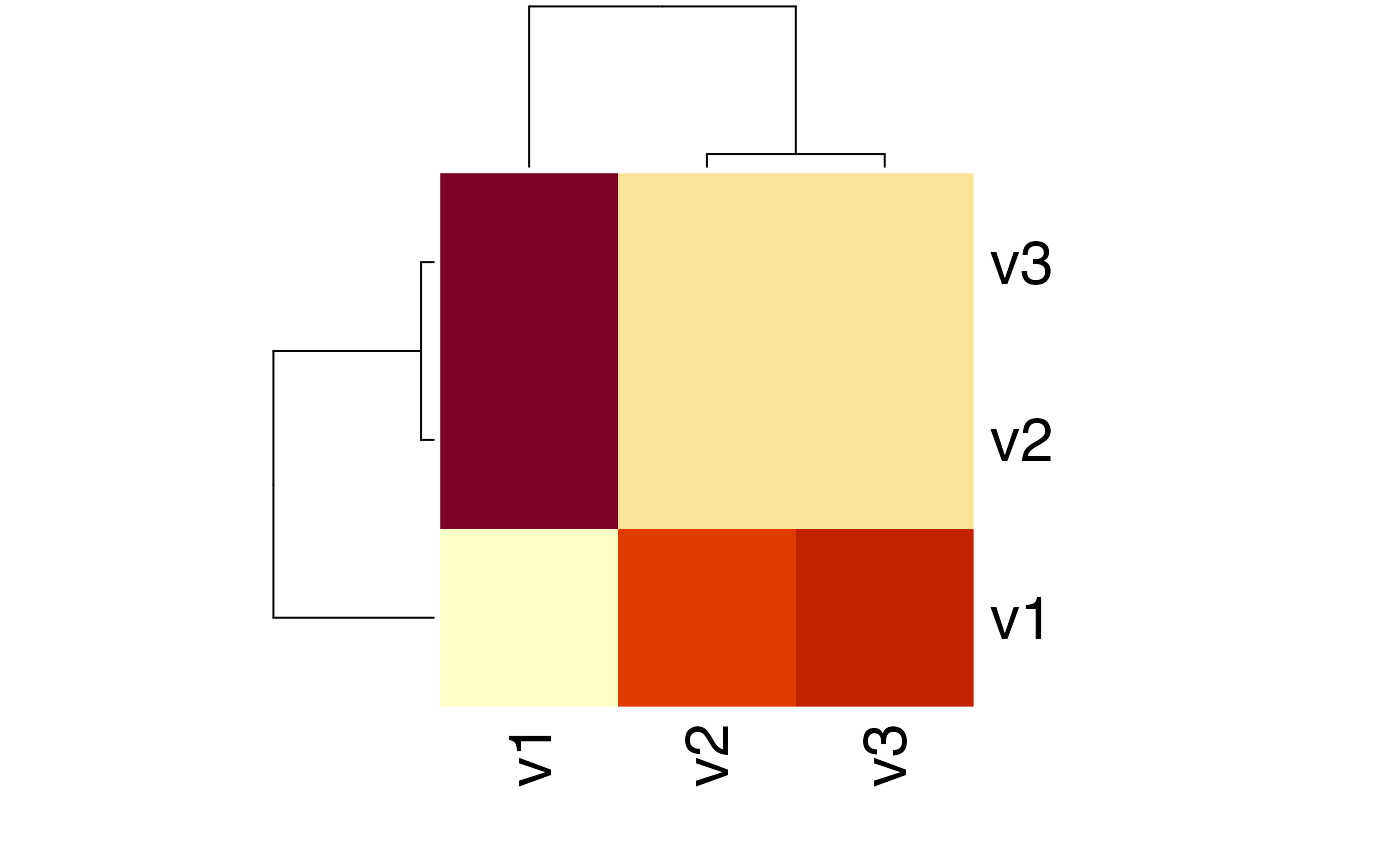This function computes the Kullback-Leibler divergence of two probability distributions P and Q.

KL(x, test.na = TRUE, unit = "log2", est.prob = NULL)

## Arguments

x a numeric data.frame or matrix (storing probability vectors) or a numeric data.frame or matrix storing counts (if est.prob = TRUE). See distance for details. a boolean value indicating whether input vectors should be tested for NA values. a character string specifying the logarithm unit that shall be used to compute distances that depend on log computations. method to estimate probabilities from a count vector. Default: est.prob = NULL.

## Value

The Kullback-Leibler divergence of probability vectors.

## Details

$$KL(P||Q) = \sum P(P) * log2(P(P) / P(Q)) = H(P,Q) - H(P)$$

where H(P,Q) denotes the joint entropy of the probability distributions P and Q and H(P) denotes the entropy of probability distribution P. In case P = Q then KL(P,Q) = 0 and in case P != Q then KL(P,Q) > 0.

The KL divergence is a non-symmetric measure of the directed divergence between two probability distributions P and Q. It only fulfills the positivity property of a distance metric.

Because of the relation KL(P||Q) = H(P,Q) - H(P), the Kullback-Leibler divergence of two probability distributions P and Q is also named Cross Entropy of two probability distributions P and Q.

Cover Thomas M. and Thomas Joy A. 2006. Elements of Information Theory. John Wiley & Sons.

H, CE, JSD, gJSD, distance

Hajk-Georg Drost

## Examples


# Kulback-Leibler Divergence between P and Q
P <- 1:10/sum(1:10)
Q <- 20:29/sum(20:29)
x <- rbind(P,Q)
KL(x)
#> Metric: 'kullback-leibler' using unit: 'log2'; comparing: 2 vectors.#> kullback-leibler
#>        0.1392629
# Kulback-Leibler Divergence between P and Q using different log bases
KL(x, unit = "log2") # Default
#> Metric: 'kullback-leibler' using unit: 'log2'; comparing: 2 vectors.#> kullback-leibler
#>        0.1392629 KL(x, unit = "log")
#> Metric: 'kullback-leibler' using unit: 'log'; comparing: 2 vectors.#> kullback-leibler
#>       0.09652967 KL(x, unit = "log10")
#> Metric: 'kullback-leibler' using unit: 'log10'; comparing: 2 vectors.#> kullback-leibler
#>        0.0419223
# Kulback-Leibler Divergence between count vectors P.count and Q.count
P.count <- 1:10
Q.count <- 20:29
x.count <- rbind(P.count,Q.count)
KL(x, est.prob = "empirical")
#> Metric: 'kullback-leibler' using unit: 'log2'; comparing: 2 vectors.#> kullback-leibler
#>        0.1392629
# Example: Distance Matrix using KL-Distance

Prob <- rbind(1:10/sum(1:10), 20:29/sum(20:29), 30:39/sum(30:39))

# compute the KL matrix of a given probability matrix
KLMatrix <- KL(Prob)
#> Metric: 'kullback-leibler' using unit: 'log2'; comparing: 3 vectors.
# plot a heatmap of the corresponding KL matrix
heatmap(KLMatrix)